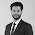What is Equilibrium

Equilibrium is when all forces on an object are equal, meaning that every force is counteracted by an equal and opposite force or that the net force is equal to zero. This is called the equilibrium rule. However, this doesn't mean that every force has to be equal.

An object in equilibrium must also have an acceleration of 0 m/s/s. It either must be at rest or in continuous motion with the same speed and direction. Static equilibrium is when an object is not in motion and in equilibrium.Two types of equilibrium are internal and external. Internal equilibrium includes the different forces that are acting within the system. External equilibrium describes the forces and reactions of the entire system. However, both equilibriums have conditions that apply to both of them, which are:

Sum of all vertical forces = 0
Sum of all horizontal forces = 0
Sum of all moments = 0

For objects that are hanging still and not in motion, the forces acting upwards on the object has to be balanced with the other forces acting downwards to equal zero. A book that is lying on a table is also in equilibrium. The support force of the table pushing up on the book balances the gravity force of the book pushing down. Usually forces that are acting in an upwards direction are positive, while forces that act downwards are typically recorded as negative.

Different Forces in Equilibrium

2 Forces:

• Equal magnitudes
• Opposite (180º)

3 Forces:

• Resultant-Equilibrant
• Choose two forces and find the result
• If the remaining force is equal and opposite of the resultant, then it is called the equilibrant
• Triangle of Forces
• If three forces can be arranged in a triangle, then they are in equilibrium

"N" Forces

• Polygon of Forces
• If three or more forces can be arranged to form a polygon, then they are in equilibrium

What is a Force

A force is a push or a pull on an object, and relies on objects interacting with each other. They are measured in Newtons (N). One Newton is equivalent to the force that is needed to give an object of 1kg mass an acceleration of 1 m/s/s. A force is also a vector quantity, meaning it has both magnitude and direction. Newtons only represent the magnitude of the force, so the direction of the force must be shown with arrows. The combination of all of the forces on an object is called the net force, and changed the motion of the object.There are two broad categories of forces: contact forces and at-a-distance forces.

Contact Forces

Contact forces are forces that occur when two objects are touching each other. Frictional forces, tensional forces, normal forces, air resistance forces, spring forces, and applied forces are all examples of contact forces.

Applied force - force that is directly applied to an object by another object

• Ex: person pushing a desk; applied force by person on the desk

Normal force - force that supports an object resting on another object
• Ex: book resting on a table; normal force by table up on book
Friction force - force that occurs when an object slides across another object
• Ex: book sliding across a table; desk's frictional force against motion of book

Air resistance force - special type of frictional force that acts on objects that move through the air
Tension force - force that is sent through a string or rope when it is pulled tightly from forces on its opposite ends

Spring force - force of a compressed or stretched spring that acts on the object attached to it

At-a-Distance Forces

At-a-distance forces are forces that occur even when the objects are not physically touching each other. Gravitational forces, electric forces, and magnetic forces are examples of at-a-distance forces.

Gravitational force - force in which a massive object attracts other objects to it (weight)

• Ex: The moon is kept in orbit with Earth because the force of gravity affects it

Electric force - force of attraction or repulsion between two charged objects

Magnetic force - force of attraction or repulsion in a magnetic field

1.1.